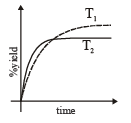Courses

# Test: MCQs (One or More Correct Option): Equilibrium | JEE Advanced

## 12 Questions MCQ Test Chemistry 35 Years JEE Main & Advanced Past year Papers | Test: MCQs (One or More Correct Option): Equilibrium | JEE Advanced

Description
This mock test of Test: MCQs (One or More Correct Option): Equilibrium | JEE Advanced for JEE helps you for every JEE entrance exam. This contains 12 Multiple Choice Questions for JEE Test: MCQs (One or More Correct Option): Equilibrium | JEE Advanced (mcq) to study with solutions a complete question bank. The solved questions answers in this Test: MCQs (One or More Correct Option): Equilibrium | JEE Advanced quiz give you a good mix of easy questions and tough questions. JEE students definitely take this Test: MCQs (One or More Correct Option): Equilibrium | JEE Advanced exercise for a better result in the exam. You can find other Test: MCQs (One or More Correct Option): Equilibrium | JEE Advanced extra questions, long questions & short questions for JEE on EduRev as well by searching above.
*Multiple options can be correct
QUESTION: 1

### For the gas phase reaction :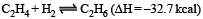carried out in a vessel, the equilibrium concentration of C2H4 can be increased by :

Solution:

The reaction is exothermic, hence increasing temperature will favour backward reaction (i.e. conc. of C2H4 increases) removing H2 and adding C2H6 favours backward reaction.

*Multiple options can be correct
QUESTION: 2

### When NaNO3 is heated in a closed vessel, oxygen is liberated and NaNO2 is left behind. At equilibrium.

Solution: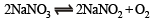According to Le-chatelier principle an increase in pressure always favours the reaction, where volume or moles decrease (i.e. reverse direction). As heat is added, i.e. reaction is endothermic and is supported in forward direction with increase in temperature.

*Multiple options can be correct
QUESTION: 3

### The equilibrium :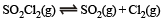is attained at 25ºC in a closed container and an inert gas, helium is introduced. Which of the following statements are correct?

Solution:

At constant volume, concentrations do not change.

*Multiple options can be correct
QUESTION: 4

For the reaction :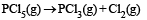The forward reaction at constant temperature is favoured by

Solution:

Introduction of an inert gas at constant pressure causes the equilibrium to shift in a direction in which number of moles increases. The forward reaction is further accelerated by increase in the quantity of substrate, i.e., PCl5 and by the increase of space, i.e., volume of container.

*Multiple options can be correct
QUESTION: 5

For the reaction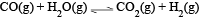at a given temperature the equilibrium amount of CO2(g) can be increased by

Solution: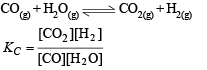A catalyst simply helps in attaining the equilibrium earlier.
Addition of inert gas has no effect on a reaction because in it Δn = 0.
This equilibrium is not based upon volume because in it  Δn = 0.
On increasing the amount of CO, KC will decrease but it is constant at constant temperature, so for maintaining the constant value of KC, the amount of CO2 increases.

*Multiple options can be correct
QUESTION: 6

Which of the following statements(s) is (are) correct?

Solution:

pH of 1 x 10-8 M is below 7 because it is an acid.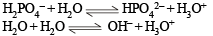K (Auto protolysis constant of water i.e. ionic product of water) increases with temperature. For half neutralisation of a weak acid by a weak base,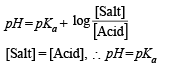*Multiple options can be correct
QUESTION: 7

A buffer solution can be prepared from a mixture of

Solution:

A buffer solution is prepared by mixing a weak acid/ base with salt of its conjugate base/acid.

*Multiple options can be correct
QUESTION: 8

Aqueous solutions of HNO3 , KOH, CH3COOH and CH3COONa of identical concentrations are provided. The pair(s) of solutions which form a buffer upon mixing is(are)

Solution:

Any solution of a weak acid and its salt with strong base acts as an acidic buffer solution.
If volume of HNO3 solution added is less as compared to that of CH3COONa solution, it results in the formation of an acidic buffer solution.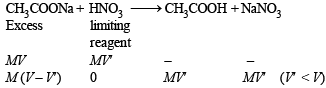*Multiple options can be correct
QUESTION: 9

The initial rate of hydrolysis of methyl acetate (1M) by a weak acid (HA, 1M) is 1/100th of that of a strong acid (HX, 1M), at 25°C. The Ka of HA is

Solution:

As ester hydrolysis is first order with respect [H+].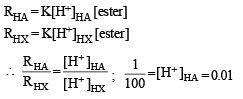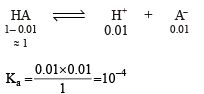*Multiple options can be correct
QUESTION: 10

The Ksp of Ag2CrO4 is 1.1 × 10–12 at 298 K. The solubility (in mol/L) of Ag2CrO4 in a 0.1 M AgNO3 solution is

Solution: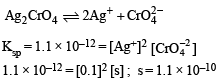*Multiple options can be correct
QUESTION: 11

The thermal dissociation equilibrium of CaCO3(s) is studied under different conditions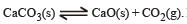For this equilibrium, the correct statement(s) is(are)

Solution: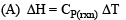Hence enthalpy depends on temperature.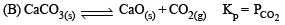(C) Keq depends only on temperature and not on Pressure.
(D) Enthalpy of reaction is independent of the catalyst.
Catalyst generaly changes activation energy.

*Multiple options can be correct
QUESTION: 12

The %yield of ammonia as a function of time in the reaction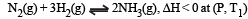is given below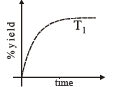If this reaction is conducted at (P, T2), with T2 > T1, the %yield of ammonia as a function of time is represented by

Solution:

Initially on increasing temperature rate of reaction will increase, so % yield will also increase with time. But at equilibrium % yield at high temperature (T2) would be less than at T1 as reaction is exothermic so the graph is